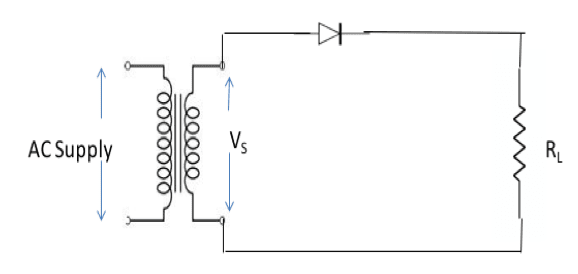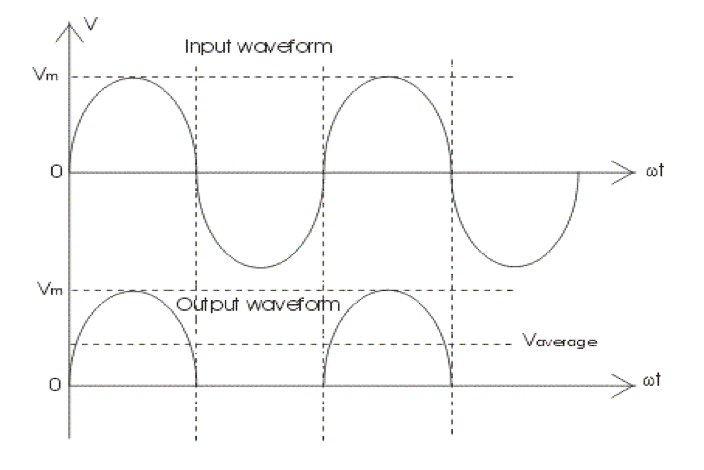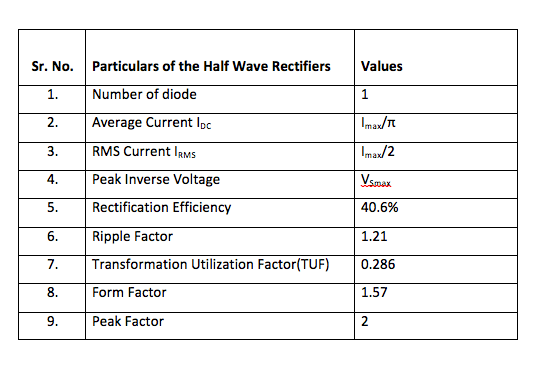Coffee Room
Discuss anything here - everything that you wish to discuss with fellow engineers.
12920 Members
Join this group to post and comment.# Half Wave Rectifiers Brief Explanation - Operation, Advantages And Disadvantages

The rectifiers are broadly classified into two types viz:

Half Wave rectifier and Full wave rectifier.

Lets discuss Half Wave rectifiers today.

Circuit of Half wave rectifier:

1. It basically consist of the diode.As we already know that the diode is the two terminal device, consisting of the cathode and the anode, thus it allows the flow of electrons in only one direction.
2. It consist of the load element RL .
3. The diode is connected in series with the secondary of the transformer and the load element RL.
4. The primary of the transformer is connected to AC mains of the supply.The half wave rectifier converts this AC voltage or alternating voltage into unidirectional pulsating voltage of half of the pulsating cycle . the other half is suppressed since the diode conducts only in one direction.

Operation:

1. We know that the AC voltage changes its polarity during every half cycle.

2. During the positive half cycle the upper end of the secondary is positive, the diode is forward biased and thus the diode conducts. And hence the current flow for that positive half cycle. This positive half cycle is then applied to the load element RL . The waveforms of the output current and voltage are of the same shape as that of the input positive wave.

Positive half ------- forward biased diode ------- output is positive half

3. During the other half i.e. negative half cycle of the AC input the upper end of the secondary is negative with respect to the lower end. Hence the diode is reversed biased. Thus during the negative half cycle, the diode does not conduct, so the current and the voltage remains zero.

Negative half ------ reversed biased diode ---------- output is Zero.

4. Thus if we see the waveform of the half wave rectifiers, its clearly seen that it conducts only for the positive half of the cycle. And for the other half the output is zero. Since is rectifies only half of the input , it is called half wave rectification.1. It’s a simple circuit easy to construct.
2. It’s very cheap.